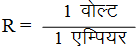Ex﻿

# 1. Electricity

#### Ohm's Law

Ohm's Law
Definition : According to the Ohm’s law at constant temperature, the current flowing through a conductor is directly proportional to the potential difference across the conductor.
Thus, if I is the current flowing through a conductor and V is the potential difference (or voltage) across the conductor, then according to Ohm’s law.
I ∝ V (when T is constant)
or, I = V/R ...(i)
where R is a constant called the resistance of the conductor.
Equation (i) may be written as, -
V = I x R ...(ii)
Unit of resistance :
The SI unit of resistance (R) is ohm. Ohm is denoted by the Greek letter omega (Ω).
From Ohm’s law, R = V/I
Now, if, V = 1 Volt and I = 1 Ampere
Then,Thus, 1 ohm is defined as the resistance of a conductor which allows a current of 1 ampere to flow through it when a potential difference of 1 volt is maintained across it.
Results of Ohm’s Law
Current flowing through a conductor is directly proportional to the potential difference across the conductor.When the potential difference in a circuit is kept constant, the current in inversely proportional to the resistance of the conductor.
I ∝ 1/R
The ratio of potential difference to the current is constant. The value of the constant is equal to the resistance of the conductor (or resistor).
V/I = R

If you want to give information about online courses to other students, then share it with more and more on Facebook, Twitter, Google Plus. The more the shares will be, the more students will benefit. The share buttons are given below for your convenience.
×

#### NTSE Physics (Class X)

• Electricity
• Magnetic Effect of Curent
• Light Reflection
• Light Refraction
• Human Eye & Colourful World
• Source of Energy
• Motion

#### NTSE Physics (Class IX)

• Force & laws of motion
• Work and Energy
• Gravitation
• Sound

SHOW CHAPTERS